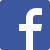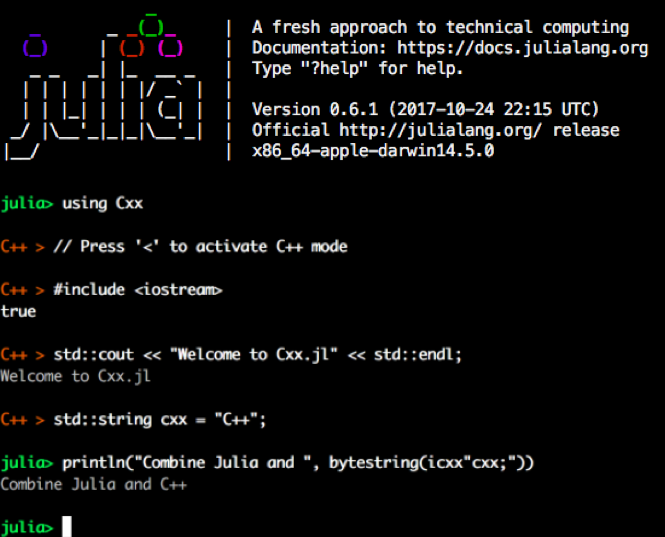Here is the latest from Julia Computing
BLG# Introduction to the Packages Cxx.jl and CxxWrap.jl

01 Dec 2017 | Keno Fischer

Cxx.jl is a Julia package that provides a C++ interoperability interface for Julia. It also provides an experimental C++ REPL mode for the Julia REPL. With Cxx.jl, it is possible to directly access C++ using the @cxx macro from Julia.

With Cxx.jl and CxxWrap.jl. when facing the task of wrapping a C++ library in a Julia package, authors now have 2 options:

• Use Cxx.jl to write the wrapper package in Julia code
• Use CxxWrap to write the wrapper completely in C++ (and one line of Julia code to load the .so)

## Cxx.jl

Functionality

There are two ways to access the main functionality provided by this package. The first is using the @cxx macro, which puns on Julia syntax to provide C++ compatibility.

The macro supports two main usages:

• A static function call @cxx mynamespace::func(args...)
• A membercall (where m is a CppPtr, CppRef or a CppValue) @cxx m->foo(args...)

Additionally, this package provides the cxx"" and icxx"" custom string literals for inputting C++ syntax directly. The two string literals are distinguished by the C++ level scope they represent.

In summary, the two approaches to embed C++ functions in Julia discussed above would look like this :

# Using @cxx (e.g.):
cxx""" void cppfunction(args){ . . .} """ => @cxx cppfunction(args)

# Using icxx (e.g.):
julia_function (args) icxx""" *code here*  """


The C++ REPL

This package contains an experimental C++ REPL feature. Using the package will automatically add a new pane to your REPL that is accessible by pressing the < key.Installation

The package is installable on Julia 0.5 and newer and is available through Julia’s package manager:

Pkg.add("Cxx")


Building the C++ code requires the same system tools necessary for building Julia from source. Further, Debian/Ubuntu users should install libedit-dev and libncurses5-dev, and RedHat/CentOS users should install libedit-devel.

Using Cxx.jl with examples

Example 1: Embedding a simple C++ function in Julia

# include headers
julia> using Cxx
julia> cxx""" #include<iostream> """

# Declare the function
julia> cxx"""
void mycppfunction() {
int z = 0;
int y = 5;
int x = 10;
z = x*y + 2;
std::cout << "The number is " << z << std::endl;
}
"""
# Convert C++ to Julia function
julia> julia_function() = @cxx mycppfunction()
julia_function (generic function with 1 method)

# Run the function
julia> julia_function()
The number is 52


Example 2: Pass numeric arguments from Julia to C++

julia> jnum = 10
10

julia> cxx"""
void printme(int x) {
std::cout << x << std::endl;
}
"""

julia> @cxx printme(jnum)
10


Example 3: Pass strings from Julia to C++

julia> cxx"""
void printme(const char *name) {
// const char* => std::string
std::string sname = name;
// print it out
std::cout << sname << std::endl;
}
"""

julia> @cxx printme(pointer("John"))
John


Example 4: Pass a Julia expression to C++

julia> cxx"""
void testJuliaPrint() {
\$:(println("\nTo end this test, press any key")::Nothing);
}
"""

julia> @cxx testJuliaPrint()
To end this test, press any key


Example 5: Embedding C++ code inside a Julia function

function playing()
for i = 1:5
icxx"""
int tellme;
std::cout<< "Please enter a number: " << std::endl;
std::cin >> tellme;
std::cout<< "\nYour number is "<< tellme << "\n" <<std::endl;
"""
end
end
playing();


## CxxWrap.jl

This package lets you write the code for the Julia wrapper in C++, and then use a one-liner on the Julia side to make the wrapped C++ library available there.

The mechanism behind this package is that functions and types are registered in C++ code that is compiled into a dynamic library. This dynamic library is then loaded into Julia, where the Julia part of this package uses the data provided through a C interface to generate functions accessible from Julia. The functions are passed to Julia either as raw function pointers (for regular C++ functions that don’t need argument or return type conversion) or std::functions (for lambda expressions and automatic conversion of arguments and return types). The Julia side of this package wraps all this into Julia methods automatically.

Installation

Like any other registered Julia package, installation completes by running the following package manager command:

Pkg.add("CxxWrap")


Features

• Support for C++ functions, member functions and lambdas
• Classes with single inheritance, using abstract base classes on the Julia side
• Trivial C++ classes can be converted to a Julia isbits immutable
• Template classes map to parametric types, for the instantiations listed in the wrapper
• Automatic wrapping of default and copy constructor (mapped to deepcopy) if defined on the wrapped C++ class
• Facilitate calling Julia functions from C++

A Hello, World example with CxxWrap.jl

Suppose we want to expose the following C++ function to Julia in a module called CppHello:

std::string greet()
{
return "hello, world";
}


Using the C++ side of CxxWrap, this can be exposed as follows:

#include "jlcxx/jlcxx.hpp"

JULIA_CPP_MODULE_BEGIN(registry)
jlcxx::Module& hello = registry.create_module("CppHello");
hello.method("greet", &greet);
JULIA_CPP_MODULE_END


Once this code is compiled into a shared library (say libhello.so) it can be used in Julia as follows:

using CxxWrap

# Load the module and generate the functions
wrap_modules(joinpath("path/to/built/lib","libhello"))
# Call greet and show the result
@show CppHello.greet()


More such examples and documentation for the package can be found here.

This post was formatted for the Julia Computing blog by Rajshekar Behar

## Recent posts

CSV Reader Benchmarks: Julia Reads CSVs 10-20x Faster than Python and R
22 Jun 2020 | Deepak Suresh
JuliaTeam v2.0 Now Available
12 Jun 2020 | Julia Computing